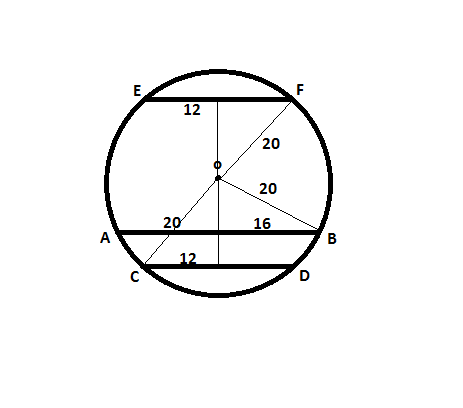0
209

Geometry is one of the most important topics in the CAT Quantitative Ability Section. You can check out these Geometry questions in CAT Previous year papers. If you want to learn the basics, you can watch these videos on Geometry basics. This article will look into some important Geometry Questions for CAT. These are good sources for practice; If you want to practice these questions, you can download this CAT Geometry Most Important Questions PDF below, which is completely Free.

Question 1: In a triangle ABC, the lengths of the sides AB and AC equal 17.5 cm and 9 cm respectively. Let D be a point on the line segment BC such that AD is perpendicular to BC. If AD = 3 cm, then what is the radius (in cm) of the circle circumscribing the triangle ABC?

a) 17.05

b) 27.85

c) 22.45

d) 32.25

e) 26.25

Solution: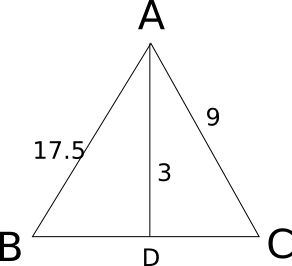Let x be the value of third side of the triangle. Now we know that Area = 17.5*9*x/(4*R), where R is circumradius.

Also Area = 0.5*x*3 .

Equating both, we have 3 = 17.5*9 / (2*R)

=> R = 26.25.

Question 2: Consider obtuse-angled triangles with sides 8 cm, 15 cm and x cm. If x is an integer then how many such triangles exist?

a) 5

b) 21

c) 10

d) 15

e) 14

Solution:

For obtuse-angles triangle, $c^2 > a^2 + b^2$ and c < a+b
If 15 is the greatest side, 8+x > 15 => x > 7 and $225 > 64 + x^2$ => $x^2$ < 161 => x <= 12
So, x = 8, 9, 10, 11, 12
If x is the greatest side, then 8 + 15 > x => x < 23
$x^2 > 225 + 64 = 289$ => x > 17
So, x = 18, 19, 20, 21, 22
So, the number of possibilities is 10

Question 3: Consider a square ABCD with midpoints E, F, G, H of AB, BC, CD and DA respectively. Let L denote the line passing through F and H. Consider points P and Q, on L and inside ABCD such that the angles APD and BQC both equal 120°. What is the ratio of the area of ABQCDP to the remaining area inside ABCD?

a) $\frac{4 \sqrt 2}{3}$

b) $2 + \sqrt{3}$

c) $\frac{10-3\sqrt{3}}{9}$

d) $1+\frac{1}{\sqrt3}$

e)  $2\sqrt{3}-1$

Solution: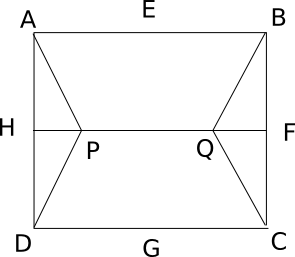Consider side of square as 10 units. So HD=5 and HP=$\frac{5}{\sqrt3}$ . So now area of triangle HPD=$\frac{12.5}{\sqrt3}$. Also Area APD=Area BQC=2*AreaHPD=$\frac{25}{\sqrt3}$. So numerator of required answer is $100-\frac{50}{\sqrt3}$ and denominator as $\frac{50}{\sqrt3}$. Solving we get answer as $2\sqrt{3}-1$.

Question 4: Two circles, both of radii 1 cm, intersect such that the circumference of each one passes through the centre of the other. What is the area (in sq. cm.) of the intersecting region?

a) $\frac{\pi}{3}-\frac{\sqrt 3}{4}$

b) $\frac{2\pi}{3}+\frac{\sqrt 3}{2}$

c) $\frac{4\pi}{3}-\frac{\sqrt 3}{2}$

d) $\frac{4\pi}{3}+\frac{\sqrt 3}{2}$

e) $\frac{2\pi}{3}-\frac{\sqrt 3}{2}$

Solution: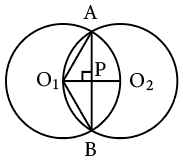The circumferences of the two circle pass through each other’s centers. Hence, O1A = O1B=O1O2 = 1cm

By symmetry, the line joining the two centres would be bisect AB and would be bisected by AB. As the line joining the center to the midpoint of a chord is perpendicular to the chord, O1O2 and AB are perpendicular bisectors of each other. Suppose they intersect at point P.

O1P = Half of O1O2 = 1/2 cm
So, the angle AO1P = 60 degrees as cos 60 = 1/2
By symmetry, BO1P = 60 degrees.
So, angle AO1B= 120 degrees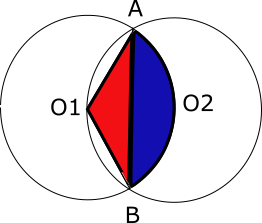In the above, the required area is 2 times A(segment ABO2)(blue region). And A(segment ABO2)(blue region) = A(sector O2AO1B)(blue + red) – A(triangleO1AB )(red)

Area of sector = 120°/360° * $\pi * 1^2$ = $\pi/3$

Area of triangle = 1/2 * b * h = 1/2 * (2* 1 cos 30°) * (1/2) = √3/4

Hence, required area = $\frac{\pi}{3}-\frac{\sqrt 3}{4}$ . Hence so the required area is 2 times the above value which is $\frac{2\pi}{3}-\frac{\sqrt 3}{2}$

Question 5: In the figure below, ABCDEF is a regular hexagon and $\angle{AOF}$ = 90° . FO is parallel to ED. What is the ratio of the area of the triangle AOF to that of the hexagon ABCDEF?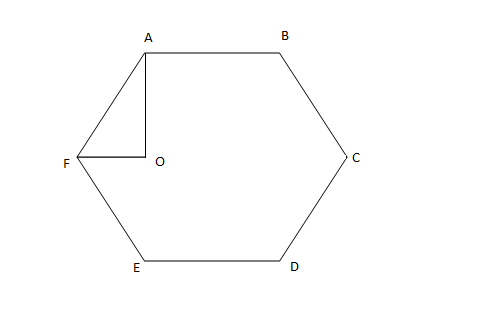a) 1/12

b) 1/6

c) 1/24

d) 1/18

Solution: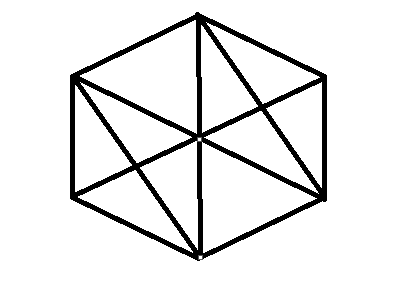When the hexagon is divided into number of similar triangle AOF we get 12 such triangles . Hence required ratio of area is 1/12.
Alternate Approach :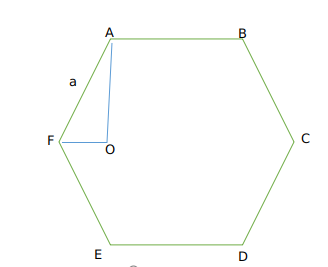Let us take side of the hexagon as a
Now We get AF = a
Now As OF || ED
Angle OFE +Angle E = 180
We know Angle E =120
So angle OFE=60 degrees.
Now therefore Angle AFO=120-60=60 degrees
Now in triangle AFO
Cos60=$\frac{OF}{AF}$
$\frac{1}{2}=\frac{OF}{AF}$
We get OF = $\frac{a}{2}$
Now therefore area of triangle AOF =$\frac{1}{2}\times\ AF\times\ OF\times\ \sin60$
So we get $\frac{1}{2}\times\ a\times\ \frac{a}{2}\times\ \frac{\sqrt{\ 3}}{2}$
=$\frac{\sqrt{\ 3}}{8}a^2$     (1)
Now area of hexagon = $6\times\ \frac{\sqrt{\ 3}}{4}a^2$      (2)
Dividing (1) and (2) we get ratio as $\frac{1}{12}$
Hence option A is the correct answer.

Checkout: CAT Free Practice Questions and Videos

Question 6: In the figure below, AB is the chord of a circle with center O. AB is extended to C such that BC = OB. The straight line CO is produced to meet the circle at D. If $\angle{ACD}$ = y degrees and $\angle{AOD}$ = x degrees such that x = ky, then the value of k is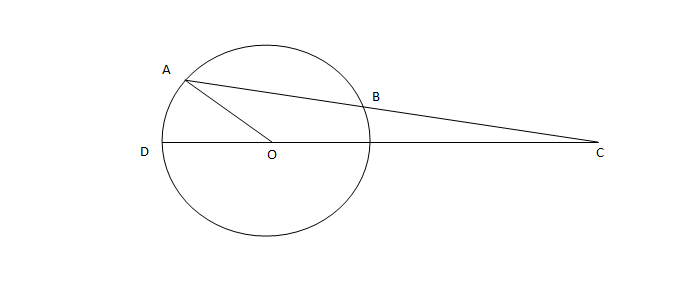a) 3

b) 2

c) 1

d) None of the above.

Solution: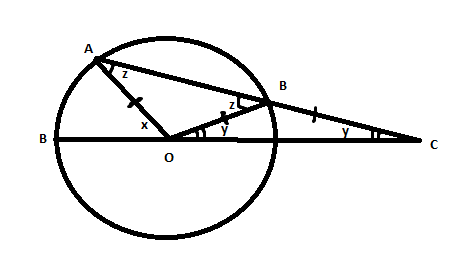Since Angle BOC = Angle BCO = y.

Angle OBC = 180-2y .

Hence Angle ABO =  z = 2y = Angle OAB.

Now since x is exterior angle of triangle AOC .

We have x = z + y = 3y.

Hence option A.

Question 7: A father and his son are waiting at a bus stop in the evening. There is a lamp post behind them. The lamp post, the father and his son stand on the same straight line. The father observes that the shadows of his head and his son’s head are incident at the same point on the ground. If the heights of the lamp post, the father and his son are 6 metres, 1.8 metres and 0.9 metres respectively, and the father is standing 2.1 metres away from the post then how far (in metres) is son standing form his father?

a) 0.9

b) 0.75

c) 0.6

d) 0.45

Solution:

The three triangles are similar.

Let the distance of the tip of the shadow from the child be y. Let the child be standing at distance x from the father.

So, 6/(2.1+x+y) = 1.8/(x+y) = 0.9/y

=> 2y = x+y => x = y

=> 6/(2.1 + 2x) = 0.9/x

=> 6x = 0.9*(2.1+2x)

=> 6x = 1.89 + 1.8x

=> 4.2x = 1.89

=> x = 1.89/4.2 = 0.45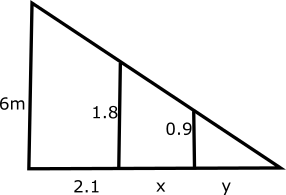Question 8: If the lengths of diagonals DF, AG and CE of the cube shown in the adjoining figure are equal to the three sides of a triangle, then the radius of the circle circumscribing that triangle will be?a) equal to the side of the cube

b) $\sqrt 3$ times the side of the cube

c) 1/$\sqrt 3$ times the side of the cube

d) impossible to find from the given information

Solution:

Consider side of the cube as x.

So diagonal will be of length $\sqrt{3}$ * x.

Now if diagonals are side of equilateral triangle we get area = 3*$\sqrt{3}*x^2$ /4 .

Also in a triangle

4 * Area * R = Product of sides

4* 3*$\sqrt{3}*x^2$ /4 * R = .3*$\sqrt{3}*x^3$

R = x

Question 9: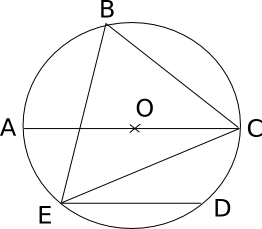In the adjoining figure, chord ED is parallel to the diameter AC of the circle. If angle CBE = 65°, then what is the value of angle DEC?

a) 35

b) 55

c) 45

d) 25

Solution: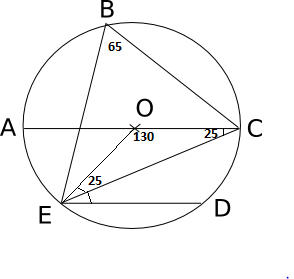If EBC = 65 then EOC = 130 then OEC = OCE = 25. NOw since OC and ED are parallel we have OCE = OED = 25. Hence option D.

Question 10: What is the distance in cm between two parallel chords of lengths 32 cm and 24 cm in a circle of radius 20 cm?

a) 1 or 7

b) 2 or 14

c) 3 or 21

d) 4 or 28